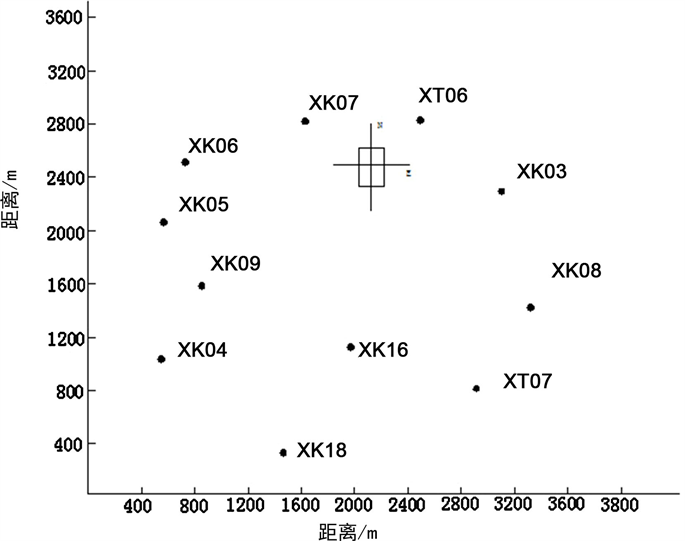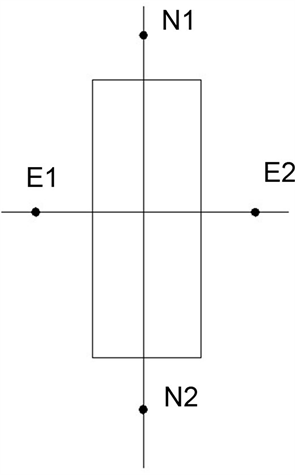# CORS-RTK在煤矿岩移观测中修正模型研究Study on CORS-RTK Correction Model in Coal Mine Rock Movement Observation

DOI: 10.12677/GST.2018.63020, PDF, HTML, XML, 下载: 670  浏览: 1,182

Abstract: CORS-RTK has been widely used in the ground survey engineering of coal mines, but its application in the monitoring of rock movement and settlement in coal mines is still insufficient. In this paper, the measurement of the normal height of rock movement observation in coal mine was discussed. And the CORS-RTK elevation correction model is constructed based on mine area quasi-geoid and the interval elevation anomaly change rate. The example analysis has obtained good accuracy and reliability, and has a certain application value.

1. 引言

CORS-RTK技术给现代矿山测量带来技术变革     。CORS-RTK技术较之传统测量技术可以提高测量精度、减少工作量、提高工作效率、节约资金等优势，已在矿山测量中获得广泛应用  -  。但，CORS-RTK测量高程成果的精度显著低于平面测量精度，特别是正常高测量精度远低于传统几何水准测量精度，大大地限制了其在煤矿岩移沉降监测上的应用   -  。

2. 修正模型

CORS-RTK测量可获得监测点平面位置及高程信息，其中高程为以大地水准面为参考面的大地高经由仪器内置模型拟合出监测点高程异常进而转换为点位正常高，大地高与正常高关系见式(1)     。但由于CORS-RTK内置转换模型通常为大范围区域似大地水准面模型，有时无法精确反应小区域内的高程异常变化趋势，故需对CORS-RTK测量中高程异常变化项进行改正。

${h}_{r}={H}_{84}-\zeta$ (1)

2.1. 高程异常变化率修正模型

${\alpha }_{ab}=\left(\Delta {H}_{ab}-\Delta {h}_{ab}\right)/{S}_{ab}$ (2)

$\Delta {\xi }_{ab}={\alpha }_{ab}{S}_{ab}$ (3)

${\stackrel{¯}{\xi }}_{j}={\xi }_{a}-\Delta {\xi }_{aj}\left({\stackrel{¯}{\xi }}_{j}={\xi }_{a}-\Delta {\xi }_{bj}\right)$ (4)

${h}_{j}={H}_{j}-{\stackrel{¯}{\xi }}_{j}$ (5)

2.2. 二次曲面拟合修正模型

${\xi }_{j}={a}_{0}+{a}_{1}{x}_{j}+{a}_{2}{y}_{j}+{a}_{3}{x}^{2}{}_{j}+{a}_{4}{y}^{3}{}_{j}+{a}_{5}{x}_{j}{y}_{j}+\Delta$ (6)

${h}_{j}={H}_{j}-{\xi }_{j}$ (7)

3. 算例及分析

3.1. 测区概况及已有数据Figure 1. Second mining area range and distribution of known point

3.2. 修正计算

1) 高程异常变化率修正计算

2) 二次曲面拟合修正计算

$\begin{array}{c}\xi =-8.785383456-\left(3.64×{10}^{-5}\right)x+\left(3.56×{10}^{-5}\right)y+\left(6.01×{10}^{-8}\right)xy\\ +\left(-4.33×{10}^{-8}\right){x}^{2}+\left(7.29×{10}^{-9}\right){y}^{2}\end{array}$ (8)Figure 2. Distribution of control points in the second mining areaTable 1. Calculation results of elevation abnormal change rate

3) 精度分析

3.3. 结果分析Table 2. Correction results of CORS-RTK rock-movement monitoring pointsTable 3. Correction results of CORS-RTK rock-movement monitoring pointsTable 4. Model Correction Accuracy

4. 结束语

CORS-RTK技术在矿山测量中具有明显技术优势，鉴于其在煤矿岩移沉降监测方面的限制，构建了利用CORS-RTK进行较高精度正常高测量修正模型。利用实例进行了验证，结果表明：在合理限制和外业观测条件良好的情况下，利用修正模型获得了测点正常高精度的明显提高，可以达到四等几何水准测量的精度。该模型可用于煤矿岩移沉降监测，具有很好的推广应用价值。

NOTES

*通讯作者。

  康请宣. CORS-RTK在矿山测量中的优缺点分析[J]. 矿业工程, 2012, 10(2): 43-45.  丁文利, 王怀念, 黄良. 动态GPS(ＲTK)测量的精度分析[J]. 地矿测绘, 2004, 20(2): 16-17.  章红平, 温宇斌, 张志勇, 等. 静态GPS测量与RTK测量实例分析[J]. 测绘通报, 2006(8): 1295-1298.  李恒宝, 郭友发. CORS-RTK技术在矿山测量中的应用[J]. 西部探矿工程, 2011, 23(6): 157-159.  王琦, 韩晓冬, 段淑珍. 基于CORS的网络-RTK矿山开采沉陷监测方法研究[J]. 测绘与空间地理信息, 2014, 37(9): 32-34.  余金富. CORS-RTK技术在矿山测量中的运用[J]. 测绘技术装备, 2016, 18(2): 82-85.  岳尊彩, 高明章, 康方, 等. CORS-RTK测量技术在兴隆庄煤矿的应用[J]. 煤矿现代化, 2015, 128(5): 86-88.  徐耀文. 宁夏CORS在矿山中的应用与实践[J]. 测绘与空间地理信息, 2013, 36(12): 238-241.  袁德宝, 崔希民, 潘星, 等. RTK实时动态测量技术在矿区测绘中的应用[J]. 大地测量与地球动力学, 2007, 27(3): 72-75.  蔡福禄. GPS-RTK技术用于开采沉陷观测试验数据处理及精度分析[J]. 矿山测量, 2010, 4(4): 31-33.  何绍福, 古玉葵, 芮昌龙. 参考站网(CORS系统)在小龙潭布沼坝露天矿的应用[J]. 露天采矿技术, 2010(5): 42-44.  朱小玉, 赵丽, 王延国, 等. CORS技术在矿区地表沉陷监测中的应用[J]. 北京测绘, 2011(4): 49-52.  张涛, 张丕亚, 魏超. 带状交通工程中GPS高程拟合方法分析[J]. 北京测绘, 2018, 32(6): 654-657.  GBT 18314-2009. 全球定位系统(GPS)测量规范[S].  魏二虎, 等. GPS测量操作与数据处理[M]. 武汉: 武汉大学出版社, 2004.